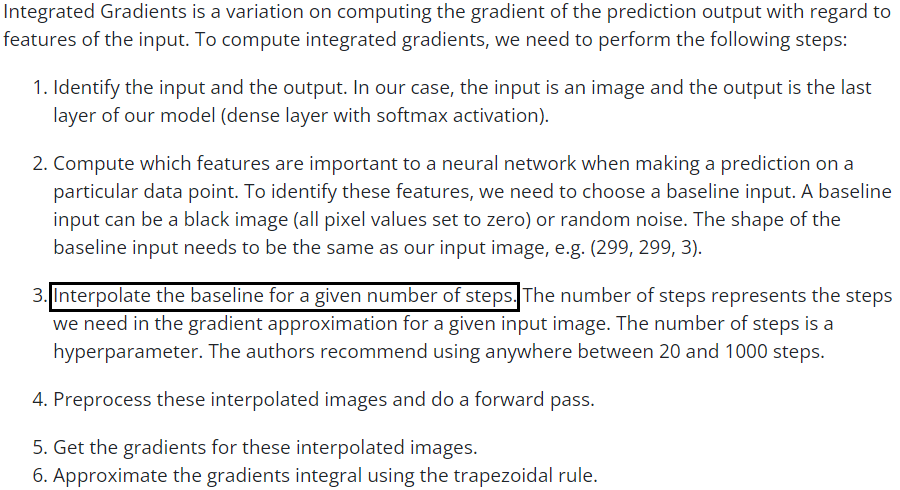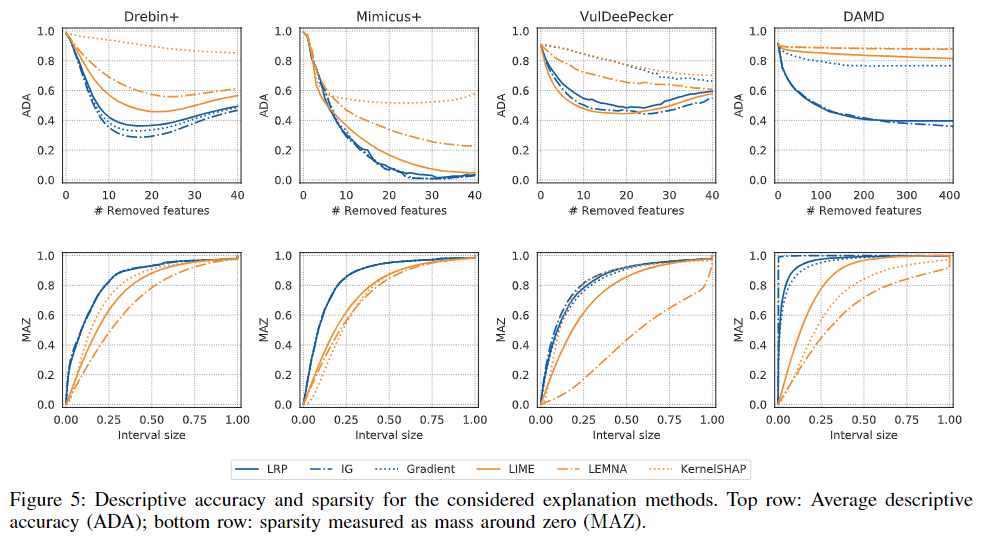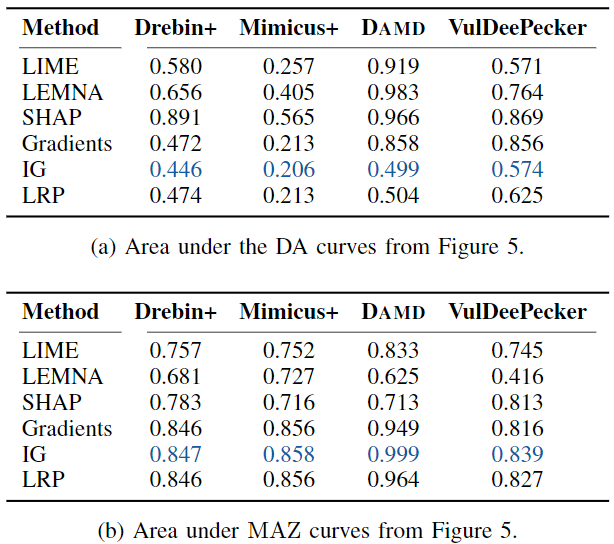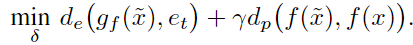# 可解释机器学习在安全中的应用和评估

Contents

## Evaluating Explanation Methods for Deep Learning in Security

EuroS&P 2020, Code available### Abstract

It is an open question which explanation methods are appropriate for computer security and what requirements they need to satisfy.

### Introduction

DL in Security: severe drawback -> lack of transparency

(Existing works)Interpreting DL: CV -> trace back the predictions to individual regions in images; received little attention in security -a single technique-> LEMNA

• 较多使用的都是较复杂的模型结构，本身就难以解释（require complex NN architectures that are challenging to investigate）
• 除了解释准确外，还需满足一些安全领域特定的需求（do not only need to be accurate but also satisfy security-specific requirements）

Provide a bridge between deep learning in security and explanation method developed for other application domains of machine learning.

• Developed evaluation criteria
• General: accuracy, sparsity -> a security practitioner cannot investigate large sets of features at once.
• Security: [completeness, stability, robustness, efficiency] -> ensure that reliable explanations are available to practitioners in all cases and in reasonable time.
• Analyze 6 explanation methods in 4 tasks

Demonstrate the utility of explainable learning -> qualitatively examine the generated explanations

• LRP: provides a nuanced representation of the relevant features 颜色都较浅
• LEMNA: unsharp explanation due to a lack of sparsity 不稀疏，都正相关
• LIME: provides an explanation that even contradicts the first one 在VAR2和VAR3上与LRP矛盾

-> highlights the need for comparing explanation methods and determining the best fit for a given security task.

(Findings) also unveils a notable number of artifacts in the underlying datasets.

features that are unrelated to security but strongly contribute to the predictions

Argue that explanation methods need to become an integral part of learning-based security systems

• first, for understanding the decision process of deep learning
• second, for eliminating artifacts in the training datasets.

## Explanation Strategies

• input vector $$x=(x_1, ..., X_d)$$ , neural network $$N$$, prediction $$f_N(x)=y$$
• explanation - why the label $$y$$ has been selected by $$N$$ -> $$r=(r_1, ..., r_d)$$ describes the relevance of the dimensions of $$x$$ for $$f_N(x)$$

relevance 值通常是实数，并且可以通过 heatmap 的形式覆盖在输入上，这样特征的相关性就能被可视化地强调出来。

• 黑盒的方法假设模型架构和参数不可知，适用场景是审计远程提供的服务，但由于缺失了模型相关的重要信息，其效果有一定的局限性；
• 白盒方法适用于独立运行的系统（如公司的恶意应用检测系统），但是一些白盒方法只对特定的网络结构有效，不具备通用性。

Adversarial example: 目标 $$f_N(x+\delta) \neq f_N(x)$$ 是为了得到最小扰动 $$\delta$$；可以通过解释方法来增强

Feature selection: 选择有区别度的特征，来减少学习时用的特征维度；研究对象是dataset，独立于学习模型而确定

### Black-box Explanation

operate under a black-box setting that assumes no knowledge about the neural network and its parameters

(Technically) rest on an approximation of the function $$f_N$$

#### LIME and SHAP

##### LIME
• approximate the local neighborhood of $$f_N$$ at the point $$f_N(x)$$ by creating a series of $$l$$ perturbations of $$x$$ -> $$\widetilde{x}_1,...,\widetilde{x}_l$$
• approximate the decision boundary by a weighted linear regression model $$\underset{g\in \mathcal{G}}{\mathrm{argmin}} \sum_{i=1}^{l}\pi_x(\widetilde{x}_i)(f_N(\widetilde{x}_i)-g(\widetilde{x}_i))^2$$，where $$\mathcal{G}$$ is the set of all linear functions and $$\pi_x$$ is a function indicating the difference between the input $$x$$ and the perturbation $$\widetilde{x}$$
##### SHAP
• uses the SHAP kernel as weighting function $$\pi_x$$ -> create Shapley Values when solving the regression

#### LEMNA

• specifically designed for security applications

• uses a mixture regression model for approximation -> a weighted sum of K linear models $$f(x)=\sum_{j=1}^K \pi_j(\beta_j \cdot x + \epsilon_j)$$

• Fused Lasso:

### White-box Explanation

can directly compute explanations for the function $$f_N$$ on the structure of the network -> usually the case for stand-alone systems for malware detection, binary analysis, and vulnerability discovery

in practice, predictions and explanations are often computed from within the same system

##### Saliency Map (simple gradients, 显著图)
• how much $$y$$ changes with respect to $$x_i$$
• $r=\partial y / \partial x_i$
• 解决的问题：真正的神经网络高度非线性，某个像素或特征增强到一定程度后可能对网络决策的贡献达到饱和。
• 例：大象的鼻子对神经网络将一个物体识别为大象的决策很重要，但当大象的鼻子长度增加到一定程度后（比如1米），继续增加不会带来决策分数的增加，导致输出对输入特征的梯度为0，即原始显著图在饱和区将其重要度设为0。积分梯度法使用沿整条梯度线的积分值，作为鼻子长度对决策分类的重要程度。
• 对于一张给定图片，大象鼻子长度已定（如2米），如何得到鼻子长度小于等于2米时输入对输出的梯度？假设当前图片为$$x$$，如果已知鼻子长度为0米时的基线图片$$x^\prime$$，则可以做线性插值：$$x^\prime+\alpha(x- x^\prime)$$。其中常数$$\alpha=0$$时，输入即基线图片；$$\alpha=1$$时，即当前图片

• $r_i=\int_0^1\frac{\partial f_N(x^\prime + \alpha(x- x^\prime))}{\partial x_i}d(\alpha(x- x^\prime))=(x-x^\prime)\int_0^1\frac{\partial f_N(x^\prime + \alpha(x- x^\prime))}{\partial x_i}d\alpha$Keras Example: Model interpretability with Integrated Gradients

#### LRP and DeepLift

##### Layer-wise Relevance Propagation (LRP)
• central idea: a conservation property that needs to hold true during the backward pass -> the relevance of the unit $$i$$ in layer $$l$$ of the neural network hold true for all $$L$$ layers $$\sum_ir_i^1=\sum_ir_i^2=...=\sum_ir_i^L$$
• $$\epsilon-LRP$$: $$R_j=\sum_k\frac{z_{jk}}{\sum_jz_{jk}}R_k=\sum_k \frac{a_jw_{jk}}{\epsilon+\sum_0^j a_jw_{jk}} R_k$$
• 其中 $$a_j$$ 表示浅层神经元 $$j$$ 的输出，$$k$$ 为相邻深层的神经元，$$w$$ 表示连接相邻层神经元间的权重，从输出层开始，一直计算到input层，对应输入特征的相关性

Tutorial: Implementing Layer-Wise Relevance Propagation

##### DeepLift
• performs a backward pass but takes a reference activation $$y^\prime=f_N(x\prime)$$ of a reference input $$x^\prime$$
• enforces the conservation law that $$\sum_ir_i=y-y\prime=\Delta y$$

## Evaluation Criteria

Do the considered explanation methods provide different results?

In light of the broad range of available explanation methods, the practitioner is in need of criteria for selecting the best methods for a security task at hand.

### General Criteria: Descriptive Accuracy

Follow an indirect strategy: measure how removing the most relevant features changes the prediction -> 实际是fidelity

• 定义 Descriptive Accuracy (DA): 对样本 $$x$$，移除 $$k$$ 个最相关的特征后，模型在原预测类别上的概率 -> $$DA_k(x, f_N)=f_N(x\vert x_1=0,...,x_k=0)c$$
• 越小说明解释越准

### General Criteria: Descriptive Sparsity

Assign high relevance to features which impact a prediction -> a human analyst can only process a limited number of the selected features.

• 定义 Mass Around Zero (MAZ): 将相关值归一化到 $$[-1,1]$$ 内，计算直方图上对称连续区间的积分 -> $$MAZ(r)=\int_{-r}^r h(x)d(x), r\in[0,1]$$
• 稀疏的解释会在 $$r$$ 接近 $$0$$ 时 MAZ 值急剧升高，而接近 $$1$$ 时趋于扁平

### Security Criteria: Completeness

Generate non-degenerated (非退化的) explanations for all possible input vectors of the prediction function $$f_N$$

• Several white-box methods are complete by definition, as they calculate relevance vectors directly from the weights of the neural network.
• For black-box methods, however, the situation is different: If a method approximates the prediction function $$f_N$$ using random perturbations, it may fail to derive a valid estimate of $$f_N$$ and return degenerated explanations.

### Security Criteria: Stability

Relevant features must not be affected by fluctuations and need to remain stable over time in order to be useful for an expert.

• 定义：The generated explanations do not vary between multiple runs -> $$IS(i,j) > 1-\epsilon$$ for some threshold $$\epsilon$$，即 intersection size 接近于1

### Security Criteria: Efficiency

Explanations need to be available in a reasonable time -> without delaying the typical workflow of an expert.

• A negative example: the runtime of LEMNA depends on the size of the inputs -> for DAMD with 530,000 features, it requires about an hour.

### Security Criteria: Robustness

Robustness of explanations to attacks that disconnect the explanation from the underlying prediction.

## Evaluation

• 白盒使用 NNvestigate toolbox-> Gradients, IG（$$N=64$$ steps，由于 VulDeePecker 嵌入层维度较高所以用的是 $$256$$；baseline使用全0吗？）, LRP（$$\epsilon=10^{-3}$$）；
• 黑盒 -> LEMNA（参照论文复现，使用 cvxpy package 解决有Fused Lasso限制的线性回归问题；$$K=3, l=500$$，Fused Lasso 中的参数 $$S$$ 对非序列的方法设为 $$10^4$$，序列方法设为 $$10^{-3}$$），LIME（$$l=500$$，使用余弦相似性度量临近性，使用 scipy package 实现有 L1正则 的线性回归），SHAP（原作开源的代码，使用 KernelSHAP solver）

### Descriptive Accuracy & Sparsity#### accuracy实验

remove特征的方法：

• 数值特征（Drebin+ 和 Mimicus+）的置零 remove features by setting the corresponding dimensions to 0.
• 序列特征的，For DAMD, we replace the most relevant instructions with the no-op opcode, and for VulDeePecker we substitute the selected tokens with an embedding-vector of zeros.

• Notably, for the DAMD dataset -> Malware Genome, IG and LRP are the only methods to generate real impact on the outcome of the classifier.

#### sparsity实验

• In case of DAMD, we see a massive peak at 0 for IG, showing that it marks almost all features as irrelevant. According to the previous experiment, however, it simultaneously provides a very good accuracy on this data. The resulting sparse and accurate explanations are particularly advantageous for a human analyst since the DAMD dataset contains samples with up to 520,000 features. The explanations from IG provide a compressed yet accurate representation of the sequences which can be inspected easily.

### Completeness of Explanations

• creating malicious perturbations from benign samples is a hard problem, especially for Drebin+ and DAMD: The problem of incomplete explanations is rooted in the imbalance of features characterizing malicious and benign data in the datasets.

In an optimal case one can achieve p ≈ 0.5, however during our experiments we find that 5%can be sufficient to calculate a non-degenerated explanation in some cases.

左图情况中，能满足 5% 扰动样本标签置反的样本只有 31%

### Stability of Explanations

• use $$k=10$$ for all datasets except for DAMD where we use $$k=50$$ due to the larger input space.
• Gradients, IG, and LRP: deterministic -> all 1.0
• none of those methods obtains a intersection size of more than 0.5. This indicates that on average half of the top features do not overlap when computing explanations on the same input. <- the output is highly dependent on the perturbations used to approximate the decision boundary.

### Efficiency of Explanations

performed on a regular server system with an Intel Xeon E5 v3 CPU at 2.6 GHz.

• Gradients and LRP achieve the highest throughput in general beating the other methods by orders of magnitude. This advantage arises from the fact that data can be processed batch-wise for methods like Gradients, IG, and LRP, that is, explanations can be calculated for a set of samples at the same time.
• Computing these methods on a GPU results in additional speedups of a factor up to three.

### Robustness of Explanations (existing literature)

#### Attack White-box

usenix’19: Interpretable Deep Learning under Fire.

The crafted input $$\widetilde{x}$$ is misclassified by the network but keeps an explanation very close to the one of $$x$$.NIPS’19: Explanations can be manipulated and geometry is to blame.

Many white-box methods can be tricked to produce an arbitrary explanation $$e_t$$ without changing the classification.#### Attack Black-box

AIES’19: Fooling lime and shap: Adversarial attacks on post hoc explanation methods.

#### difficult to assess

• access to specific parts of the victim system: white->the model parameters, black->pass the classification process of the perturbations

• further extenions to work in discrete domains: while binary features, as in the Drebin+ dataset, require larger changes with $$\vert δ \vert ≥1$$. Similarly, for VulDeePecker and DAMD,a direct application of existing attacks will likely result in broken code or invalid behavior.

## Insights

### Drebin+

• several benign applications are characterized by the hardware feature touchscreen, the intent filter launcher, and the permission INTERNET. These features frequently occur in benign and malicious applications in the Drebin+ dataset and are not particularly descriptive for benignity. -> conclude that the three features together form an artifact in the dataset that provides an indicator for detecting benign applications. (这个结论不太行，本身设计的特征就是与恶意行为相关的，有解释benign的必要吗？)
• For malicious Android applications, the situation is different: The explanation methods return highly relevant features that can be linked to the functionality of the malware. For instance, the requested permission SEND_SMS  or features related to accessing sensitive information, such as the permission READ_PHONE_STATE and the API call getSimCountryIso, receive consistently high scores in our investigartion. These features are well in line with common malware for Android, such as the FakeInstaller family, which is known to obtain money from victims by secretly sending text messages (SMS) to premium services. -> Our analysis shows that the MLP network employed in Drebin+ has captured indicative features directly related to the underlying malicious activities. (符合所有malware无意义)

### VulDeePecker

still difficult for a human analyst to benefit from the highlighted tokens:

• an analyst interprets the source code rather than the extracted tokens and thus maintains a different view on the data.
• truncates essential parts of the code. In Figure 4, during the initialization of the destination buffer, for instance, only the size remains as part of the input.
• the large amount of highlighted tokens like semicolons, brackets, and equality signs seems to indicate that VulDeePecker overfits to the training data at hand.

conclude that the VulDeePecker system might benefit from

• extending the learning strategy to longer sequences
• cleansing the training data to remove artifacts that are irrelevant for vulnerability discovery.

### DAMDIn this function, the malware intercepts incoming SMS and phone calls and stores the information in local files before sending them to an external server.
Updated on